Courses

# Solutions of Refraction of Light (Page No- 247) - Physics By Lakhmir Singh, Class 10 Class 10 Notes | EduRev

## Class 10 : Solutions of Refraction of Light (Page No- 247) - Physics By Lakhmir Singh, Class 10 Class 10 Notes | EduRev

The document Solutions of Refraction of Light (Page No- 247) - Physics By Lakhmir Singh, Class 10 Class 10 Notes | EduRev is a part of the Class 10 Course Class 10 Physics Solutions By Lakhmir Singh & Manjit Kaur.
All you need of Class 10 at this link: Class 10

Lakhmir Singh Physics Class 10 Solutions Page No:247

Question 15: A converging lens of focal length 5 cm is placed at a distance of 20 cm from a screen. How far from the lens should an object be placed so as to form its real image on the screen ?

Solution :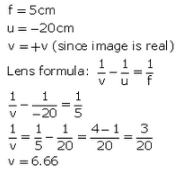Question 16: An object 5 cm high is held 25 cm away from a converging lens of focal length 10 cm. Find the position, size and nature of the image formed. Also draw the ray diagram.

Solution :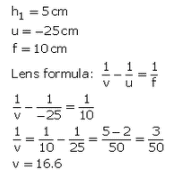Image is 16.6cm behind the oonvex lens.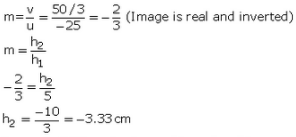Image is 3.33 cm in size and Is real and inverted.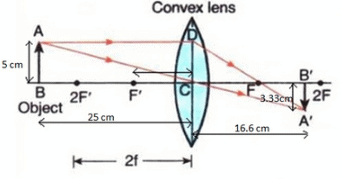Question 17: At what distance should an object be placed from a convex lens of focal length 18 cm to obtain an image at 24 cm from it on the other side ? What will be the magnification produced in this case ?

Solution :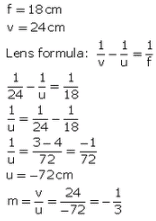Question 18: An object 2 cm tall is placed on the axis of a convex lens of focal length 5 cm at a distance of 10 m from the optical centre of the lens. Find the nature, position and size of the image formed. Which case of image formation by convex lenses is illustrated by this example ?

Solution :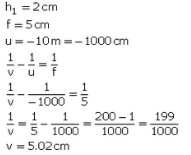The image is formed 5.02cmbehind die convex lens and is real and inverted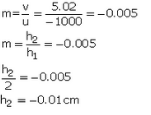Since the object distance is much greater than the focal length, this example illustrates the case when the object is place

Question 19: The filament of a lamp is 80 cm from a screen and a converging lens forms an image of it on a screen, magnified three times. Find the distance of the lens from the filament and the focal length of the lens.

Solution : -u + v = 80cm------(1)
m = -3 (The image is real, since it firms en a screen)
m = v/u = -3
v = -3u

Put in eq (1),
u = -3u = 80
-4u = 80
u = -20cm
Distance of lens from filament is 20cm.
v = -3u = 60cm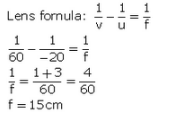Question 20: An erect image 2.0 cm high is formed 12 cm from a lens, the object being 0.5 cm high. Find the focal length of the lens.

Solution :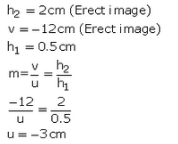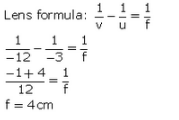Question 21: A convex lens of focal length 0.10 m is used to form a magnified image of an object of height 5 mm placed at a distance of 0.08 m from the lens. Calculate the position, nature and size of the image.

Solution :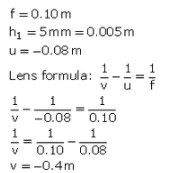Image is formed 0.40 m in front of the convex lens.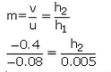h2 = 0.025m = 25mm
Size of image is 25 mm.
Image is virtual and erect

Question 22: A convex lens of focal length 6 cm is held 4 cm from a newspaper which has print 0.5 cm high. By calculation, determine the size and nature of the image produced.

Solution :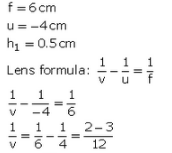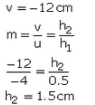Image is 1.5 cm high, virtual, erect and magnified.

Question 23: Determine how far an object must be placed in front of a converging lens of focal length 10 cm in order to produce an erect (upright) image of linear magnification 4.

Solution :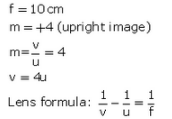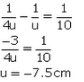The object must be placed 7.5 cm in front of the converging lens.

Question 24: A lens of focal length 20 cm is used to produce a ten times magnified image of a film slide on a screen. How far must the slide be placed from the lens ?

Solution :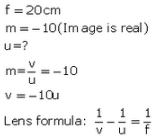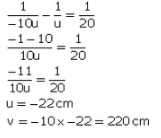Question 25: An object placed 4 cm in front of a converging lens produces a real image 12 cm from the lens.
(a) What is the magnification of the image ?
(b) What is the focal length of the lens ?
(c) Draw a ray diagram to show the formation of image. Mark clearly F and 2F in the diagram.

Solution :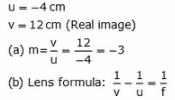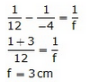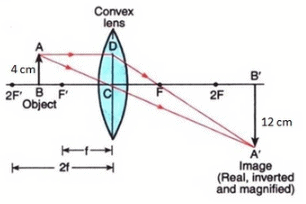Question 26: (a) An object 2 cm tall stands on the principal axis of a converging lens of focal length 8 cm. Find the position, nature and size of the image formed if the object is :
(i) 12 cm from the lens
(ii) 6 cm from the lens
(b) State one practical application each of the use of such a lens with the object in position (i) and (ii).

Solution :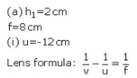Image is 24 cm behind the lens.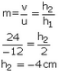Image is 4 an high, real and inverted,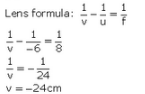Image is 24 cm in front of the lens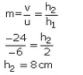Image is 8 cm high, virtual and erect.
(b) (i) Used in film projectcr.
(ii) Used as a magnifying glass.

Question 27: (a) An object 3 cm high is placed 24 cm away from a convex lens of focal length 8 cm. Find by calculations, the position, height and nature of the image.
(b) If the object is moved to a point only 3 cm away from the lens, what is the new position, height and nature of the image ?
(c) Which of the above two cases illustrates the working of a magnifying glass ?

Solution :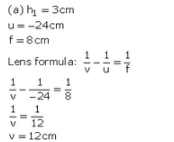Image is formed 12 cm behind the lens.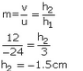Image is l.Scm high, rea! and inverted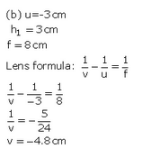Image is famed 4.8 an In front of ttie lens.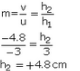Image is 4.8cm high, virtual and erect

(c) Case (b)

Question 28: (a) Find the nature, position and magnification of the images formed by a convex lens of focal length 0.20 m if the object is placed at a distance of :
(i) 0.50 m
(ii) 0.25 m
(iii) 0.15 m
(b) Which of the above cases represents the use of convex lens in a film projector, in a camera, and as a magnifying glass ?

Solution :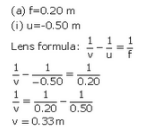Image is formed 0.33 m behind the lens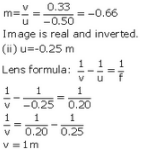Image is formed 1 m behind tfie lens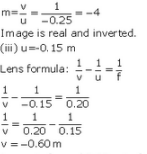Image is formed 0.60 m in front of die lens.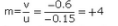Image is virtual and erect
(b) Film projector Case (ii)
Camera: Case (i)
Magnifying glass: Case (hi)

Offer running on EduRev: Apply code STAYHOME200 to get INR 200 off on our premium plan EduRev Infinity!

94 docs

,

,

,

,

,

,

,

,

,

,

,

,

,

,

,

,

,

,

,

,

,

,

,

,

;